# 树莓派-简单入门

## 一、型号

（1）Raspberry Pi 3代 B 型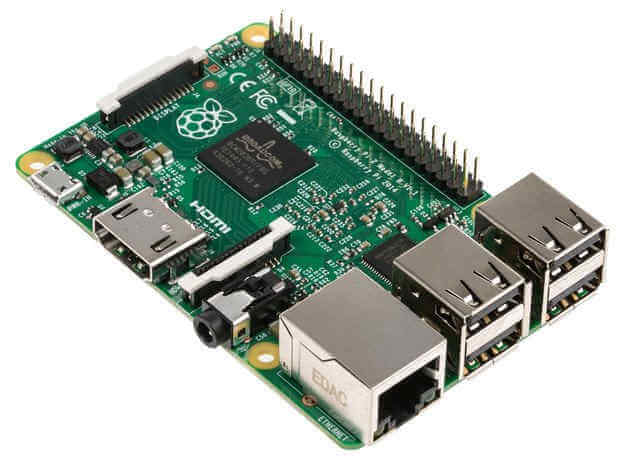（2）Raspberry Pi zero （含 zero w）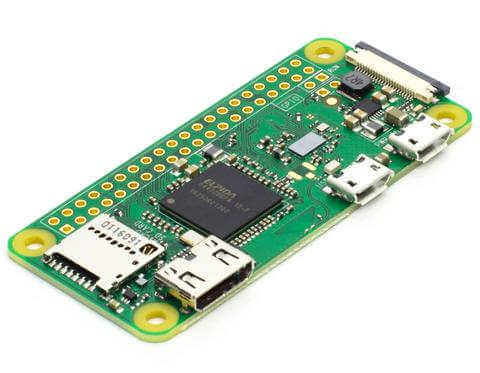## 二、配件

（1）电源

Micro USB 接口的手机充电器，就可以充当电源，但输出必须是 5V 电压、至少 2A 电流。充电宝当电源也没问题。（2）Micro SD 卡（3）显示器（4）无线键鼠## 三、电子元件

（1）面包板（一块）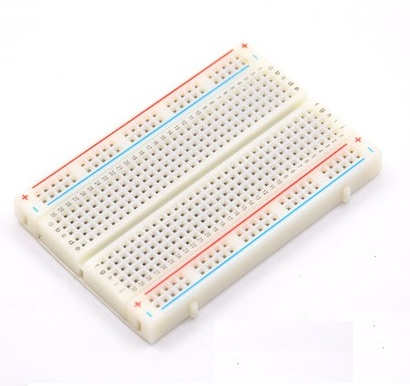（2）连接线（若干）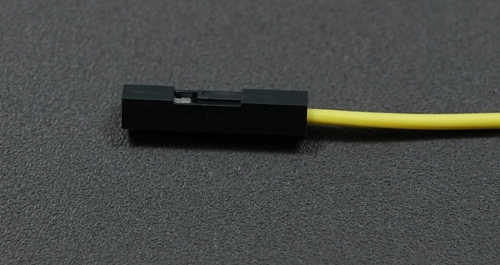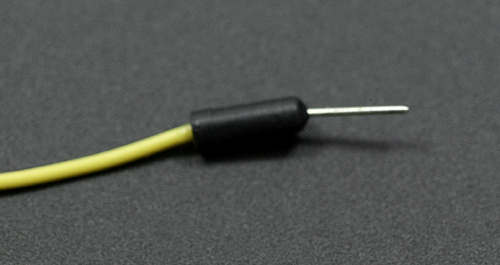（3）LED 二极管（若干）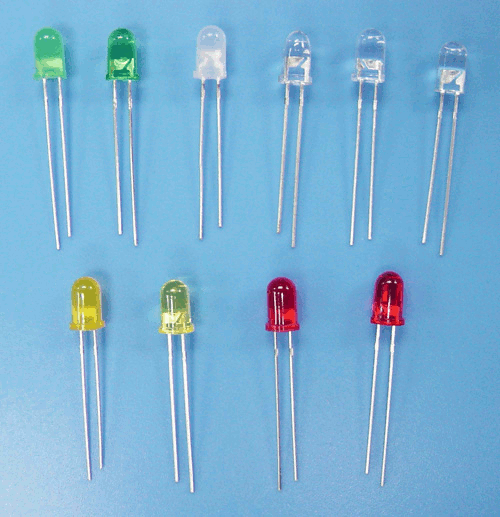（4）270欧姆的电阻（若干）## 四、安装系统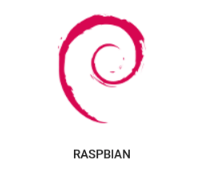```下载 NOOBS。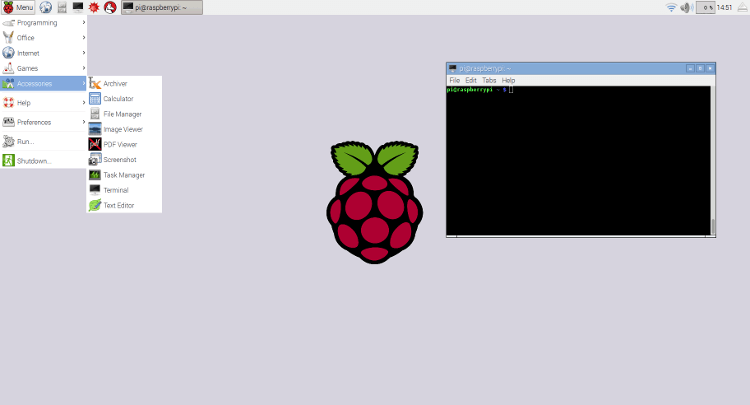## 五、SSH 登录

```\$ sudo ifconfig
```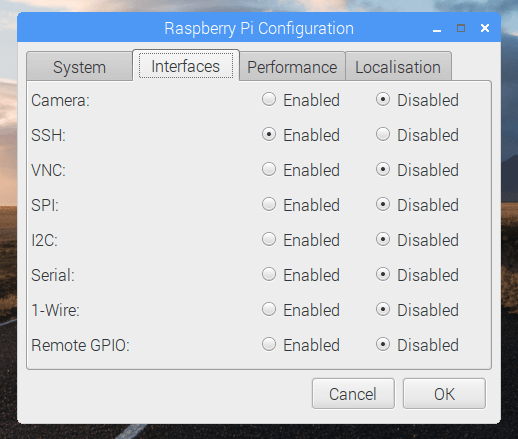```\$ ssh [email protected]
```

```\$ passwd
```

```\$ sudo adduser pi gpio
```

## 六、安装 Node

```\$ curl -sL https://deb.nodesource.com/setup_8.x | sudo -E bash -
\$ sudo apt install nodejs```

```\$ node -v
v8.1.0```

## 七、点亮 LED

### GPIO基本介绍

GPIO（General Purpose I/O Ports）意思为通用输入/输出端口，通俗地说，就是一些引脚，可以通过它们输出高低电平或者通过它们读入引脚的状态-是高电平或是低电平。GPIO是个比较重要的概念，用户可以通过GPIO口和硬件进行数据交互(如UART)，控制硬件工作(如LED、蜂鸣器等),读取硬件的工作状态信号（如中断信号）等。GPIO口的使用非常广泛。掌握了GPIO，差不多相当于掌握了操作硬件的能力。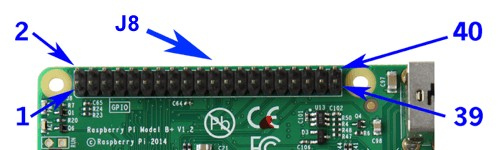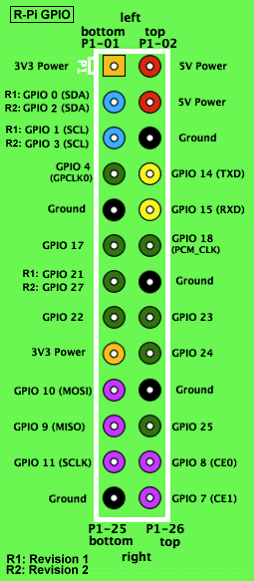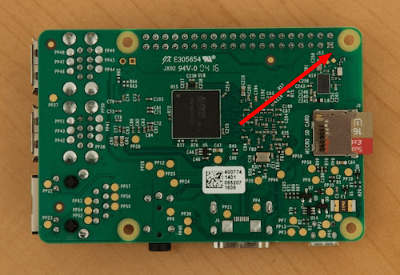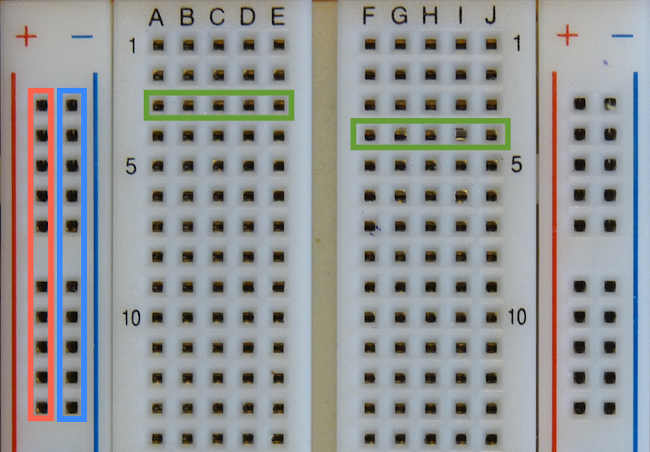`+`极和`-`极是两根垂直的导线，标着`1``5``10`这些数字的行，每一行都是一根水平的导线。导线与导线之间互不连接，另外，面包板的左右两半也是互不连接的。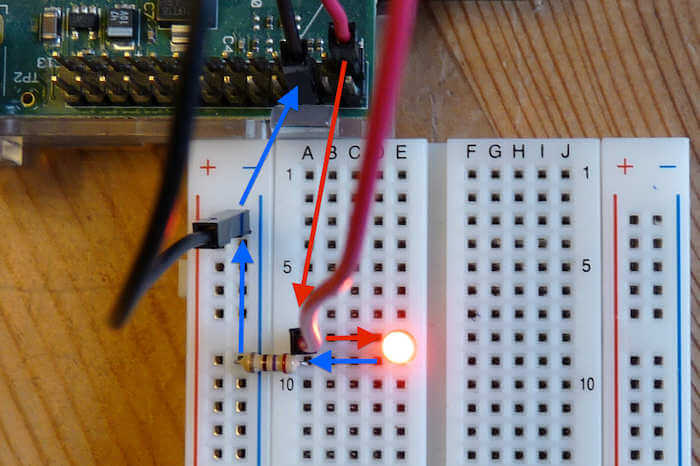## 八、LED 控制脚本

```\$ mkdir led-demo && cd led-demo
\$ npm init -y
\$ npm install -S rpio```

```// led-on.js
var rpio = require('rpio');

// 打开 11 号针脚（GPIO17) 作为输出
rpio.open(11, rpio.OUTPUT);

// 指定 11 号针脚输出电流（HIGH）
rpio.write(11, rpio.HIGH);```

```\$ node led-on.js
```

```var rpio = require('rpio');

// Configure pin 11 (GPIO17) for output (i.e. read/write).
// rpio.setOutput(11);
rpio.open(11, rpio.OUTPUT);

// 指定 11 号针脚停止输出电流（LOW）
// Turn GPIO17 off, also known as 'LOW'.
rpio.write(11, rpio.LOW);
```

```\$ node led-off.js
```

```var rpio = require('rpio');

rpio.open(11, rpio.OUTPUT);

/*
* Blink the LED quickly (10 times per second).  It is switched on every
* 100ms, and a timeout is set for 50ms later to switch it off, giving us
*/
rpio.write(11, rpio.HIGH);
setTimeout(function ledoff() {
rpio.write(11, rpio.LOW);
}, 50);
}, 100);```

```\$ node led-blink.js
```

## 九、HTTP 服务器

```\$ npm install -S server
```

```var server = require('server');
var { get } = server.router;

var rpio = require('rpio');

rpio.open(11, rpio.OUTPUT);

rpio.write(11, rpio.HIGH);
setTimeout(function ledoff() {
rpio.write(11, rpio.LOW);
}, 50);
}

server({ port: 8080 }, [
get('/' ,  ctx => {
console.log('a request is coming...');
}),
]);

console.log('server starts on 8080 port');
```

```\$ node server.js
```

```\$ curl http://localhost:8080
```

## python控制GPIO（教程可能是树莓派2的引脚，自己注意一下）

### 导入RPi.GPIO模块

```import RPi.GPIO as GPIO
```

```try:
import RPi.GPIO as GPIO
except RuntimeError:
print("引入错误")```

### 针脚编号

```GPIO.setmode(GPIO.BOARD)
# or
GPIO.setmode(GPIO.BCM)```

```mode = GPIO.getmode()
```

### 警告

```GPIO.setwarnings(False)
```

### 引脚设置

```# 将引脚设置为输入模式
GPIO.setup(channel, GPIO.IN)

# 将引脚设置为输出模式
GPIO.setup(channel, GPIO.OUT)

# 为输出的引脚设置默认值
GPIO.setup(channel, GPIO.OUT, initial=GPIO.HIGH)```

### 释放

```GPIO.cleanup()
```

### 输出

```GPIO.output(channel, state)
```

```chan_list = [11,12]
GPIO.output(chan_list, GPIO.LOW)
GPIO.output(chan_list, (GPIO.HIGH, GPIO.LOW))```

```GPIO.output(12, not GPIO.input(12))
```

### 读取

```GPIO.input(channel)
```

```GPIO.setup(channel, GPIO.IN, pull_up_down=GPIO.PUD_UP)
# or
GPIO.setup(channel, GPIO.IN, pull_up_down=GPIO.PUD_DOWN)
```

#### 轮询方式

```while GPIO.input(channel) == GPIO.LOW:
time.sleep(0.01)  # wait 10 ms to give CPU chance to do other things```

#### 边缘检测

• wait_for_edge() 函数。
wait_for_edge()被用于阻止程序的继续执行，直到检测到一个边沿。也就是说，上文中等待按钮按下的实例可以改写为
```channel = GPIO.wait_for_edge(channel, GPIO_RISING, timeout=5000)
if channel is None:
print('Timeout occurred')
else:
print('Edge detected on channel', channel)```
该函数对一个引脚进行监听，一旦引脚输入状态发生了改变，调用event_detected()函数会返回true，如下代码：
```GPIO.add_event_detect(channel, GPIO.RISING)  # add rising edge detection on a channel
do_something()
// 下面的代码放在一个线程循环执行。
if GPIO.event_detected(channel):
print('Button pressed')
```

```def my_callback(channel):
print('This is a edge event callback function!')
print('Edge detected on channel %s'%channel)

```

```def my_callback_one(channel):
print('Callback one')

def my_callback_two(channel):
print('Callback two')

### 综合例子：点亮LED灯

• 编写代码之前，首先你需要将led灯的针脚通过杜邦线连接到树莓派的引脚上，比如你可以连接到11号引脚。
• 新建一个main.py文件，写入如下代码：
```import RPi.GPIO as GPIO  //引入函数库
import time

RPi.GPIO.setmode(GPIO.BOARD)  //设置引脚编号规则
RPi.GPIO.setup(11, RPi.GPIO.OUT)    //将11号引脚设置成输出模式

while True
GPIO.output(channel, 1)   //将引脚的状态设置为高电平，此时LED亮了
time.sleep(1)   //程序休眠1秒钟，让LED亮1秒
GPIO.output(channel, 0)   //将引脚状态设置为低电平，此时LED灭了
time.sleep(1)   //程序休眠1秒钟，让LED灭1秒

GPIO.cleanup()    //程序的最后别忘记清除所有资源
```
• 保存，并退出文件。执行`python3 main.py`，即可观看效果。`Ctrl+C`可以关闭程序。
• 此外，不妨也试试其它的函数吧，增强印象。

### 使用PWM

```import time
import RPi.GPIO as GPIO   //引入库
GPIO.setmode(GPIO.BOARD)  //设置编号方式
GPIO.setup(12, GPIO.OUT)  //设置12号引脚为输出模式

p = GPIO.PWM(12, 50)  //将12号引脚初始化为PWM实例 ，频率为50Hz
p.start(0)    //开始脉宽调制，参数范围为： (0.0 <= dc <= 100.0)
try:
while 1:
for dc in range(0, 101, 5):
p.ChangeDutyCycle(dc)   //修改占空比 参数范围为： (0.0 <= dc <= 100.0)
time.sleep(0.1)
for dc in range(100, -1, -5):
p.ChangeDutyCycle(dc)
time.sleep(0.1)
except KeyboardInterrupt:
pass
p.stop()    //停止输出PWM波
GPIO.cleanup()    //清除
```

## 结语

http://www.ruanyifeng.com/blog/2017/06/raspberry-pi-tutorial.html

http://blog.hyesheng.com/2016-09-01-raspberry02/